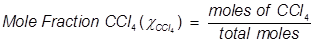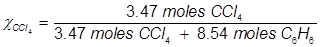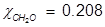# Mole Fraction Formula

Mole Fraction Formula

Mole fraction is another way of expressing the concentration of a solution or mixture. It is equal to the moles of one component divided by the total moles in the solution or mixture.a = the component that is being identified for mole fraction

Mole fraction is used in a variety of calculations, but most notably for calculating partial pressures.

Mole Fraction Formula Questions:

1. What is the mole fraction of carbon tetrachloride (CCl4) in solution if 3.47 moles of CCl4 is dissolved in 8.54 moles of benzene (C6H6)?2. What is the mole fraction of formaldehyde (CH2O) in solution if 25.7 grams of CH2O is dissolved in 3.25 moles of carbon tetrachloride (CCl4)?

The grams of acetone will need to be converted into moles in order to solve for mole fraction.Related Links: Unit Vector Formula Mole-Mass Conversions Quiz Mole Ratios Quiz Mole Facts